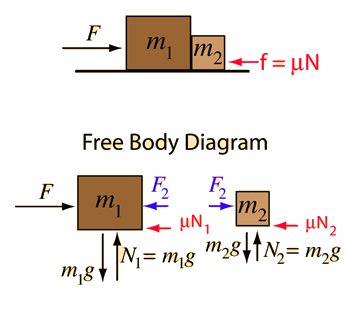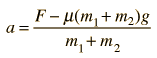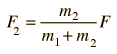# Force on Two MassesApplication of Newton's second law to two masses.
A force F= N is applied to two masses m1 =kg and m2 =kg which are in contact with each other. If there is a coefficient of friction mu = , then the net force on them is N and the acceleration is given bya = m/s²
Then the force exerted on mass m2 can be determined.= N
Note that the share of the force necessary to accelerate the second mass is independent of the friction coefficient if the coefficient of friction is the same for the two blocks, which has been assumed here.

### Remove friction

Index

Newton's laws

Standard mechanics problems

 HyperPhysics***** Mechanics R Nave
Go Back